# Plugin Points on Sphere

### General

After downloading the plugin , simple copy the file spherepoint.hxt into the HXT directory your A:M installation .The plugin is only just for fun , and therefor raises far no claim to completness or accuracy, however it is freeware .

### Description

In the a:m forum I have read a question about distributing points on a sphere from goodfrey . I found that is a problem , which are very interesting for me . So I have played with some solutions for this , and make this plugin just for fun for me .
In this plugin, I have implemented 2 solutions.
-Electrical Repulse
calculates distribution of points on sphere, which gives a minimum to 1/r potential energy
very fast , but not exact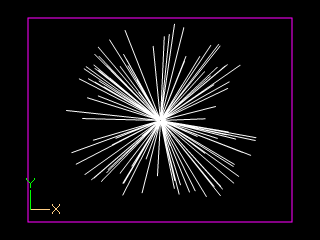Demonstrationmovie for this solution, the biggest changes are only at the begin from the calculation

-Brute force
implemented with the closed point method (moving the closed points on the sphere , until the difference becomes no longer smaller)
slow, but exacter results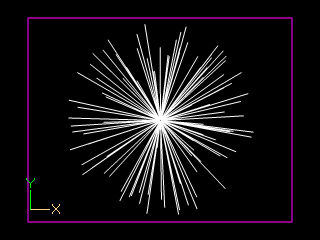Demonstrationsmovie for this method

There are also other solutions for this problem , more you can find here and here .

### Useage

Select a named group in the modelling mode and than RMB->Plug-ins->Wizards->Points on sphere starts the plugin .
The group should be independent , and have a small patch count, because she would duplicated and the duplicates would moved at the succesfully end from th plugin .
(number of patches = patchcount selected group * number of points)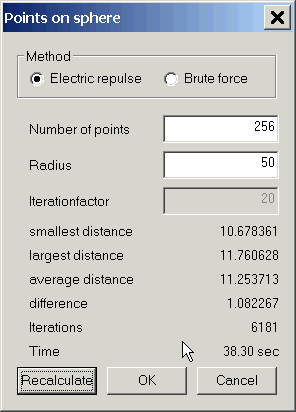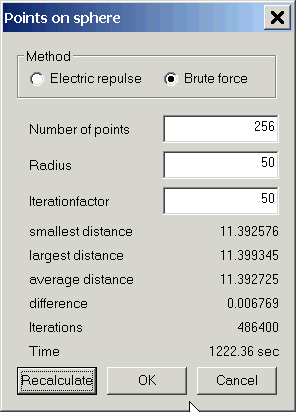Explanation concerning the individual dialogue elements
• Method
• select the calculation method
• Number of points
• number of points, which are to be distributed on the sphere
• Iterationfactor
• only for Brute force, thus it is determined how many shifting procedures for each run are made (Iterationfactor * number of points * 6)
• Calculate/Recalculate
• starting the calculation , you can break the calculation with the escape key
• Ok
• only if OK is pressed, the group is duplicated and the dupes on its new position shifted (= = change of the model)
• Cancel
• end the plugin without changing the model
Some statusfield, they would be updated at the calculation
• smallest distance
• the smallest distance between 2 closed points
• largest distance
• the largest distance between 2 closed points
• average distance
• the average distance between 2 closed points for alle points
• difference
• diefference between the largest and smallest distance (the smaller the value, the more exact is the result)
• Iterations
• number of calculations
• Time
• how long the calculation runs (the samples are calculated on a AMD64 3200+ )

Here a finished example (Projectfile)
The small yellow sphere in the center, is the group, which was duplicated .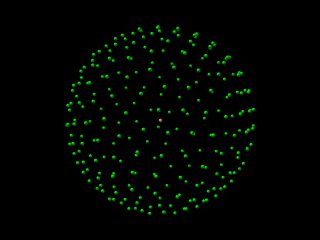Points 256, Radius 50, Iterationfaktor 50, Brute force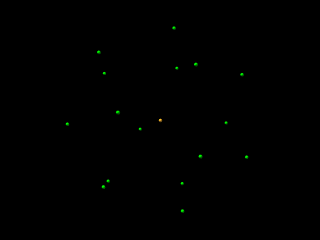Points 16, Radius 50, Iterationfaktor 1500, Brute force

Goto Top

### History

• Version 0.1
• Initialrelease

Goto Top

To the Pluginindex